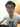# 不 evil 的 eval - Realms

August 29, 2019

``````var g = window
var r = new Realm()
var f = Realm.evaluate('(function() { return 17 })')

f() === 17 // true

Reflect.getPrototypeOf(f) === Reflect.getPrototypeOf(g) // false
Reflect.getPrototypeOf(f) === Reflect.getPrototypeOf(r) // true``````

``Realm.evaluate('log(1+1)', {log: console.log}) // print 2``Written by Yi-Shan, Chen. Github